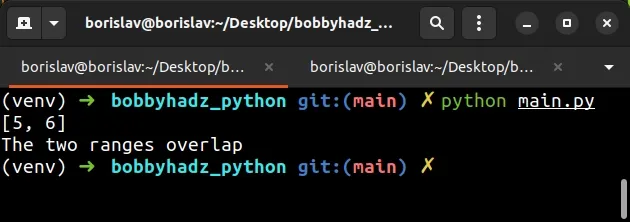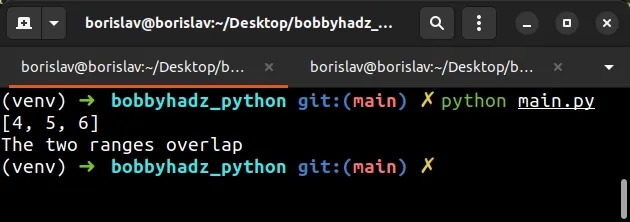# Python: Find range overlap and Check if Ranges overlapLast updated: Jun 9, 2023
4 min## #Python: Find range overlap and Check if Ranges overlap

To find the overlap between two ranges in Python:

1. Use the `max()` function to find the max starting number of the two ranges.
2. Use the `min()` function to find the min ending number of the two ranges and add `1` to the result.
3. Use the `range()` class to create a range of overlapping numbers.
main.py
```Copied!```def find_range_overlap(a, b):
return list(range(max(a, b), min(a[-1], b[-1]) + 1))

x = range(1, 7)
y = range(5, 10)

overlap = find_range_overlap(x, y)
print(overlap)  # 👉️ [5, 6]

if len(overlap) > 0:
print('The two ranges overlap')
else:
print('The two ranges do NOT overlap')
``````The `find_range_overlap()` function takes 2 ranges - `a` and `b` and returns a list containing the overlapping numbers.

We used the max() function to get the max starting value of the two ranges.

main.py
```Copied!```def find_range_overlap(a, b):
return list(range(max(a, b), min(a[-1], b[-1]) + 1))
``````

The `max()` function returns the largest item in an iterable or the largest of two or more arguments.

main.py
```Copied!```print(max(1, 5)) # 👉️ 5
``````

We then used the `min()` function to get the min number between the `stop` values of the ranges.

The min function returns the smallest item in an iterable or the smallest of two or more arguments.

Python indices are zero-based so the first item in a sequence has an index of `0` and the last item has an index of `len(sequence) - 1` or simply `-1`.

Notice that we added `1` to the min value in the call to the `range()` class.

main.py
```Copied!```def find_range_overlap(a, b):
return list(range(max(a, b), min(a[-1], b[-1]) + 1))
``````

This is necessary because the `stop` parameter of the `range()` class is exclusive (up to, but not including).

The range class is commonly used for looping a specific number of times in for loops and takes the following arguments:

NameDescription
`start`An integer representing the start of the range (defaults to `0`)
`stop`Go up to, but not including the provided integer
`step`Range will consist of every N numbers from `start` to `stop` (defaults to `1`)

The last step is to use the list class to convert the `range` object to a list.

The list contains the overlapping numbers between the supplied ranges.

main.py
```Copied!```def find_range_overlap(a, b):
return list(range(max(a, b), min(a[-1], b[-1]) + 1))

x = range(1, 7)
y = range(5, 10)

overlap = find_range_overlap(x, y)
print(overlap)  # 👉️ [5, 6]
``````

## #Checking if two ranges overlap in Python

To check if two ranges overlap:

1. Find the overlap between the ranges and store the result in a list.
2. Check if the list's length is greater than `0`.
3. If the condition is met, the two ranges overlap.
main.py
```Copied!```def find_range_overlap(a, b):
return list(range(max(a, b), min(a[-1], b[-1]) + 1))

x = range(2, 7)
y = range(4, 12)

overlap = find_range_overlap(x, y)
print(overlap)  # 👉️ [4, 5, 6]

if len(overlap) > 0:
# 👇️ this runs
print('The two ranges overlap')
else:
print('The two ranges do NOT overlap')
``````We first find the overlap between the two ranges using the `find_range_overlap` function.

The next step is to check if the list's length is greater than `0`.

If the condition is met, then the ranges overlap.

If the two ranges don't overlap, the list will be empty.

main.py
```Copied!```def find_range_overlap(a, b):
return list(range(max(a, b), min(a[-1], b[-1]) + 1))

x = range(2, 7)
y = range(10, 15)

overlap = find_range_overlap(x, y)
print(overlap)  # 👉️ []

if len(overlap) > 0:
print('The two ranges overlap')
else:
# 👇️ this runs
print('The two ranges do NOT overlap')
``````The ranges in the example above don't overlap, so the list is empty and the `else` block runs.

## #Find the overlap between two ranges using Set intersection

You can also use the `intersection` method of the `Set` object to find the overlap between two ranges.

main.py
```Copied!```def find_range_overlap(a, b):
return list(set(a).intersection(b))

x = range(2, 10)
y = range(7, 15)

overlap = find_range_overlap(x, y)
print(overlap)  # 👉️ [8, 9, 7]
``````

We used the `set()` class to convert the first `range` object to a `set` to be able to use the `intersection()` method.

The intersection method returns a new `set` with elements common to both `set` objects.

The last step is to optionally convert the new `set` to a `list`.

The `intersection()` method can be passed any iterable value so we didn't have to convert the second `range` object to a `set`.

You can also use the ampersand `&` operator which is an implicit way to call the `intersection()` method.

main.py
```Copied!```def find_range_overlap(a, b):
return list(set(a) & set(b))

x = range(2, 10)
y = range(7, 15)

overlap = find_range_overlap(x, y)
print(overlap)  # 👉️ [8, 9, 7]
``````

However, when using the `&` operator, you have to convert both `range` object to sets.

Set objects are an unordered collection of unique elements.

Therefore, this approach shouldn't be used if you need to preserve the order of the overlapping numbers.

It should also be noted that this approach is about 2.5 times slower than the one covered in the first subheading.